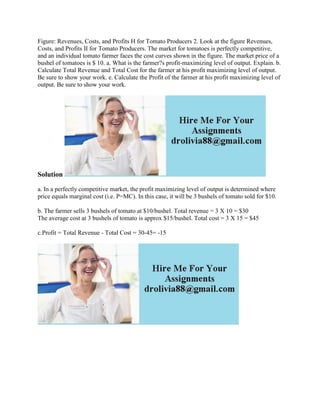Diese Präsentation wurde erfolgreich gemeldet.

# Figure- Revenues- Costs- and Profits H for Tomato Producers 2- Look.docx

Anzeige
Anzeige
Anzeige
Anzeige
Anzeige
Anzeige
Anzeige
Anzeige
Anzeige
Anzeige
Anzeige×

1 von 1 Anzeige

# Figure- Revenues- Costs- and Profits H for Tomato Producers 2- Look.docx

Figure: Revenues, Costs, and Profits H for Tomato Producers 2. Look at the figure Revenues, Costs, and Profits II for Tomato Producers. The market for tomatoes is perfectly competitive, and an individual tomato farmer faces the cost curves shown in the figure. The market price of a bushel of tomatoes is \$ 10. a. What is the farmer?s profit-maximizing level of output. Explain. b. Calculate Total Revenue and Total Cost for the farmer at his profit maximizing level of output. Be sure to show your work. e. Calculate the Profit of the farmer at his profit maximizing level of output. Be sure to show your work.
Solution
a. In a perfectly competitive market, the profit maximizing level of output is determined where price equals marginal cost (i.e. P=MC). In this case, it will be 3 bushels of tomato sold for \$10.
b. The farmer sells 3 bushels of tomato at \$10/bushel. Total revenue = 3 X 10 = \$30
The average cost at 3 bushels of tomato is approx \$15/bushel. Total cost = 3 X 15 = \$45
c.Profit = Total Revenue - Total Cost = 30-45= -15
.

Figure: Revenues, Costs, and Profits H for Tomato Producers 2. Look at the figure Revenues, Costs, and Profits II for Tomato Producers. The market for tomatoes is perfectly competitive, and an individual tomato farmer faces the cost curves shown in the figure. The market price of a bushel of tomatoes is \$ 10. a. What is the farmer?s profit-maximizing level of output. Explain. b. Calculate Total Revenue and Total Cost for the farmer at his profit maximizing level of output. Be sure to show your work. e. Calculate the Profit of the farmer at his profit maximizing level of output. Be sure to show your work.
Solution
a. In a perfectly competitive market, the profit maximizing level of output is determined where price equals marginal cost (i.e. P=MC). In this case, it will be 3 bushels of tomato sold for \$10.
b. The farmer sells 3 bushels of tomato at \$10/bushel. Total revenue = 3 X 10 = \$30
The average cost at 3 bushels of tomato is approx \$15/bushel. Total cost = 3 X 15 = \$45
c.Profit = Total Revenue - Total Cost = 30-45= -15
.

Anzeige
Anzeige

### Figure- Revenues- Costs- and Profits H for Tomato Producers 2- Look.docx

1. 1. Figure: Revenues, Costs, and Profits H for Tomato Producers 2. Look at the figure Revenues, Costs, and Profits II for Tomato Producers. The market for tomatoes is perfectly competitive, and an individual tomato farmer faces the cost curves shown in the figure. The market price of a bushel of tomatoes is \$ 10. a. What is the farmer?s profit-maximizing level of output. Explain. b. Calculate Total Revenue and Total Cost for the farmer at his profit maximizing level of output. Be sure to show your work. e. Calculate the Profit of the farmer at his profit maximizing level of output. Be sure to show your work. Solution a. In a perfectly competitive market, the profit maximizing level of output is determined where price equals marginal cost (i.e. P=MC). In this case, it will be 3 bushels of tomato sold for \$10. b. The farmer sells 3 bushels of tomato at \$10/bushel. Total revenue = 3 X 10 = \$30 The average cost at 3 bushels of tomato is approx \$15/bushel. Total cost = 3 X 15 = \$45 c.Profit = Total Revenue - Total Cost = 30-45= -15